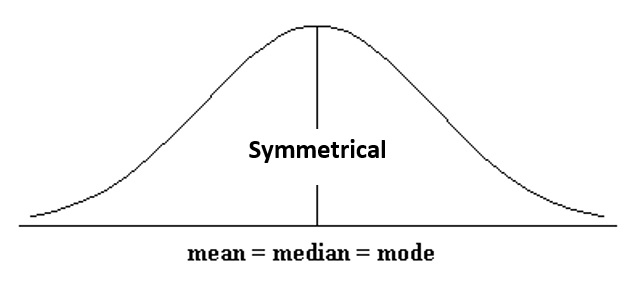# 2.2.4 - Measures of Central Tendency

2.2.4 - Measures of Central Tendency

Quantitative variables are often summarized using numbers to communicate their central tendency. The mean, median, and mode are three of the most commonly used measures of central tendency.

Mean

The numerical average; calculated as the sum of all of the data values divided by the number of values.

The sample mean is represented as $\overline{x}$ ("x-bar") and the population mean is denoted as the Greek letter $\mu$ ("mu"). The formula is the same for the sample mean and the population mean.

Population Mean
$\mu=\dfrac{\Sigma x}{N}$
Sample Mean
$\overline {x} = \dfrac{\Sigma x}{n}$
Median
The middle of the distribution that has been ordered from smallest to largest; for distributions with an even number of values, this is the mean of the two middle values.
Mode
The most frequently occurring value(s) in the distribution, may be used with quantitative or categorical variables.

## Example: Hours Spent StudyingA professor asks a sample of 7 students how many hours they spent studying for the final. Their responses are: 5, 7, 8, 9, 9, 11, and 13.

Mean

$\overline{x} = \dfrac{\sum x}{n} =\dfrac{5+7+8+9+9+11+13}{7} =\dfrac{62}{7} =8.857$

The mean is 8.857 hours.

Median

The observations are already in order from smallest to largest. The middle observation is 9 hours. The median is 9 hours.

Mode

The most frequently occurring observation was 9 hours. The mode is 9 hours.

In this example, the mean, median, and mode are all similar. Recall from our discussion of shape, the mean, median, and mode are all equal when a distribution is symmetric. This distribution of hours spent studying is probably close to symmetrical.

## Example: Test Scores

A teacher wants to examine students’ test scores. Their scores are: 74, 88, 78, 90, 94, 90, 84, 90, 98, and 80.

Mean

$\overline{x}\: =\: \dfrac{\sum x}{n} = \dfrac{74+88+78+90+94+90+84+90+98+80}{10} = \dfrac{866}{10}=86.6$

The mean score was 86.6.

Median

First, we need to put the scores in order from lowest to highest: 74, 78, 80, 84, 88, 90, 90, 90, 94, 98

Because there is an even number of scores, the median will be the mean of the middle two values. The middle two values are 88 and 90. $\frac{88+90}{2}=89$

The median is 89.

Mode

The most frequently occurring score was 90. There were 3 students who scored a 90; this is the mode. Because this distribution has one mode, it is unimodal.

In this example the mean is slightly lower than the median which is slightly lower than the mode. Recall from our discussion of shape that this occurs when a distribution is skewed to the left. This distribution is probably slightly skewed to the left.

## Example: Household Size

A group of children are asked how many people live in their household. The following data is collected: 4, 3, 6, 2, 2, 4, 3.

Mean

$\overline{x} = \dfrac{\sum x}{n}=\dfrac{4+3+6+2+2+4+3}{7}=\dfrac{24}{7}=3.429$

The mean household size in this group of children is 3.429 people.

Median

First, we need to put all of the values in order from smallest to largest: 2, 2, 3, 3, 4, 4, 6

The value in the middle of this distribution is 3. The median is 3.

Mode

In this distribution, the most common values are 2, 3, and 4. Each of these values occurs twice. There are 3 modes: 2, 3, and 4. This distribution is multimodal.

# 2.2.4.1 - Skewness & Central Tendency

2.2.4.1 - Skewness & Central Tendency

The preferred measure of central tendency often depends on the shape of the distribution. In a symmetrical distribution, the mean, median, and mode are all equal. In these cases, the mean is often the preferred measure of central tendency.For distributions that are strongly skewed or have outliers, the median is often the most appropriate measure of central tendency because in skewed distributions the mean is pulled out toward the tail. The median is more resistant to outliers compared to the mean. Of these three measures of central tendency, the mean is most influenced by outliers. Below you will see how the direction of skewness impacts the order of the mean, median, and mode.Link ↥ Has Tooltip/Popover Toggleable Visibility